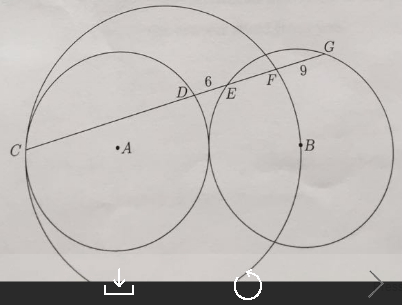Circle USAMO
2015

Problem - 2215

In the diagram below, the circle with center $A$ is congruent to and tangent to the circle with center $B$. A third circle is tangent to the circle with center $A$ at point $C$ and passes through point $B$. Points $C, A$, and $B$ are collinear. The line segment $\overline{CDEFG}$ intersects the circles at the indicated points. Suppose that $DE=6$ and $FG=9$. Find $AG$.